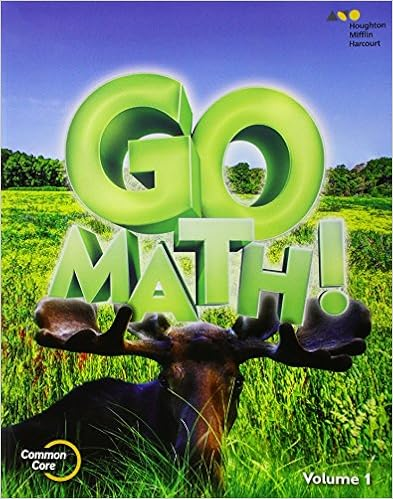•Math

GO Math! is an interactive math program that supports the Standards for Mathematical Practice by providing 3rd grade instruction in:

• Addition and Subtraction within 1,000
• Represent and Interpret Data
• Understand Multiplication
• Multiplication Facts and Strategies
• Understand Division
• Division Facts and Strategies
• Understand Fractions
• Compare Fractions
• Time, Length, Liquid Volume, and Mass
• Perimeter and Area
• Two Dimensional Shapes